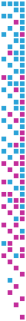# Tensor Completion for Estimating Missing Values in Visual Data

Ji Liu, Przemyslaw Musialski, Peter Wonka, Jieping Ye
Tensor Completion for Estimating Missing Values in Visual Data
IEEE Transactions on Pattern Analysis & Machine Intelligence (PAMI), 35(1):208-220, January 2012.

## Information

• Publication Type: Journal Paper (without talk)
• Workgroup(s)/Project(s):
• Date: January 2012
• ISSN: 0162-8828
• Journal: IEEE Transactions on Pattern Analysis & Machine Intelligence (PAMI)
• Number: 1
• Pages (from): 208
• Pages (to): 220
• Volume: 35
• Keywords: matrix completion, trace norm, tensor completion

## Abstract

In this paper we propose an algorithm to estimate missing values in tensors of visual data. Our methodology is built on recent studies about matrix completion using the matrix trace norm. The contribution of our paper is to extend the matrix case to the tensor case by proposing the first definition of the trace norm for tensors and then by building a working algorithm. First, we propose a definition for the tensor trace norm, that generalizes the established definition of the matrix trace norm. Second, similar to matrix completion, the tensor completion is formulated as a convex optimization problem. We developed three algorithms: SiLRTC, FaLRTC, and HaLRTC. The SiLRTC algorithm is simple to implement and employs a relaxation technique to separate the dependant relationships and uses the block coordinate descent (BCD) method to achieve a globally optimal solution; The FaLRTC algorithm utilizes a smoothing scheme to transform the original nonsmooth problem into a smooth one; The HaLRTC algorithm applies the alternating direction method of multipliers (ADMM) to our problem. Our experiments show potential applications of our algorithms and the quantitative evaluation indicates that our methods are more accurate and robust than heuristic approaches.

## BibTeX

```@article{liu-2012-tcvd,
title =      "Tensor Completion for Estimating Missing Values in Visual
Data",
author =     "Ji Liu and Przemyslaw Musialski and Peter Wonka and Jieping
Ye",
year =       "2012",
abstract =   "In this paper we propose an algorithm to estimate missing
values in tensors of visual data. Our methodology is built
on recent studies about matrix completion using the matrix
trace norm. The contribution of our paper is to extend the
matrix case to the tensor case by proposing the first
definition of the trace norm for tensors and then by
building a working algorithm. First, we propose a definition
for the tensor trace norm, that generalizes the established
definition of the matrix trace norm. Second, similar to
matrix completion, the tensor completion is formulated as a
convex optimization problem. We developed three algorithms:
SiLRTC, FaLRTC, and HaLRTC. The SiLRTC algorithm is simple
to implement and employs a relaxation technique to separate
the dependant relationships and uses the block coordinate
descent (BCD) method to achieve a globally optimal solution;
The FaLRTC algorithm utilizes a smoothing scheme to
transform the original nonsmooth problem into a smooth one;
The HaLRTC algorithm applies the alternating direction
method of multipliers (ADMM) to our problem. Our experiments
show potential applications of our algorithms and the
quantitative evaluation indicates that our methods are more
accurate and robust than heuristic approaches.",
month =      jan,
issn =       "0162-8828",
journal =    "IEEE Transactions on Pattern Analysis & Machine Intelligence
(PAMI)",
number =     "1",
volume =     "35",
pages =      "208--220",
keywords =   "matrix completion, trace norm, tensor completion",
URL =        "https://www.cg.tuwien.ac.at/research/publications/2012/liu-2012-tcvd/",
}
```